# Asymptotic power series

An asymptotic series with respect to the sequence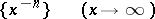or with respect to a sequence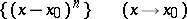(cf. Asymptotic expansion of a function). Asymptotic power series may be added, multiplied, divided and integrated just like convergent power series.

Let two functionsand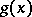have the following asymptotic expansions as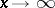: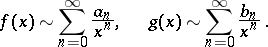Then

1)(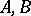are constants);

2)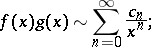3)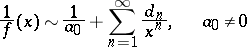(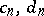are calculated as for convergent power series);

4) if the function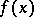is continuous for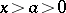, then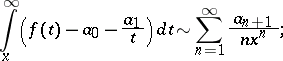5) an asymptotic power series cannot always be differentiated, but ifhas a continuous derivative which can be expanded into an asymptotic power series, then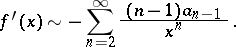Examples of asymptotic power series.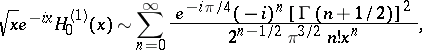whereis the Hankel function of order zero (cf. Hankel functions) (the above asymptotic power series diverge for all).

Similar assertions are also valid for functions of a complex variableas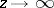in a neighbourhood of the point at infinity or inside an angle. For a complex variable 5) takes the following form: If the functionis regular in the domain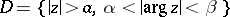and if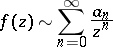uniformly inas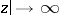inside any closed angle contained in, then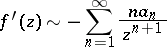uniformly in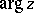asin any closed angle contained in D.